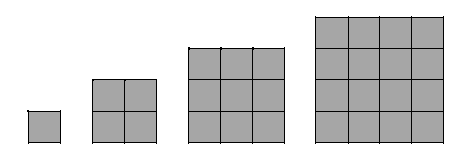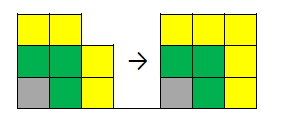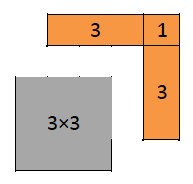# Oh Math!Mathematics used to be the subject from school days where we LEARN how to sum, we MEMORIZE the multiplication table, we ADAPT to the calculator, we are CHALLENGED by real life problems, we are told to IMAGINE inexistent and seemingly useless things. We get rid of it and then, one day, it hits us back when you less expect.

Mathematics is a way to see the world, to write down our experience, to communicate our ideas and to solve our own problems. We pass it from generation to generation. In the end, it is not that different from a culture. Our knowledge of it EVOLVES as a part of our culture in an objective way. Therefore, it is part of us… So, embrace it with a smile on your face.

As everything we produce, mathematics has the right to ELEGANCE (sometimes it can be noisy and ugly, too). Any idea is born from OBSERVATION, just like in Arts. For instance, odd numbers pass by us every day – when we walk and count the steps, we notice that if we start on the left foot, we will always hit the odd number of steps with the left foot. But have you tried to sum the first n odd numbers? As an example, if n=5, you get the sum of the first five odd numbers: 1+3+5+7+9 = 25. You try it and you will quickly notice that the result is simply n² (in our example, you get 5² = 25). For the interested ones, Why? is the next question. (An answer will be given at the end.)

However, a flawed mathematical thought, as much as a flawed argument in a discussion, can be DECEPTIVE. Those who remember someone doing 3/4 + 2/3 = 5/7 (which is, of course, incorrect) know what I mean. In a more visual example, observe the following triangles. What happened to that missing square?!  (Again, the curious ones please bear with me to the end.)

Mathematics might seem cold at the beginning, but it has the power to bring us feelings and emotions, varying from satisfaction to disillusionment (remember that being afraid of it also counts). With and in it, we LEARN, MEMORIZE, ADAPT, get CHALLENGED, IMAGINE, and EVOLVE. It involves ELENGANCE, OBSERVATION, and DECEPTION. These qualities are not unique to Mathematics.So, does Mathematics exist in our universe? Or is it just a product of our imagination? Are we the only species conscious of these notions? If yes, what do we have in our brain that makes us so different? [For these questions, unfortunately (or maybe fortunately), there are no answers at the end of this text!]

#### The sum of the first odd numbersFor those who survived all those questions, here is the answer I promised you. Remember that n² is just the area of a square with sides of length n. Then you can represent for n=1, 2, 3 and 4 as above.Now take the square of n=1. To build a bigger square of n=2 (see below), choose two adjacent sides of that square and append one green square on each side. Then add a green one on the corner. In total, you added 3 squares (2×1 (the side ones) plus 1 (the corner)) to get a bigger square of n=2. So you have 1 + 3 = 4 = 2².If you take now this square of n=2 and add two yellow squares on each of the two adjacent sides as before and one in the corner, you will get a bigger square of n=3 by adding five yellow squares to the square of n=2. So you have 1 + 3 + 5 = 3² (the grey square plus the green ones plus the yellow ones).When you repeat that step by appending in “L” shape 7 squares (the orange ones below), then 9 squares, and so on, you will successively get squares of n=4, 5, and so on, respectively!Actually, the “L” shape missing to complete a square with side length n to one with n+1 contains exactly the nth odd number of squares! [For those really interested, you can write the nth odd number as 2n+1. Picture below show that for the case of n=3. A similar idea can be applied to show that the sum of the first n positive integers is simply n(n+1)/2.]

#### The case of the missing square

Actually, if you pay (really) close attention, you will notice that none of the forms composed by the smaller ones are actually a big triangle. In fact, the “hypotenuse” of the left one is slightly convex, that is, it has a little bump, while the “hypotenuse” of the one on the right side is slightly concave. That difference of area accounts exactly for the area of that missing square!

[For the brave ones, you can check that the composed forms are not triangular. If the big form was a triangle, then its hypotenuse would be equal to the sum of the hypotenuses of the green and red triangles, which is false in both situations. You can calculate these values using the old Pythagorean Theorem.]